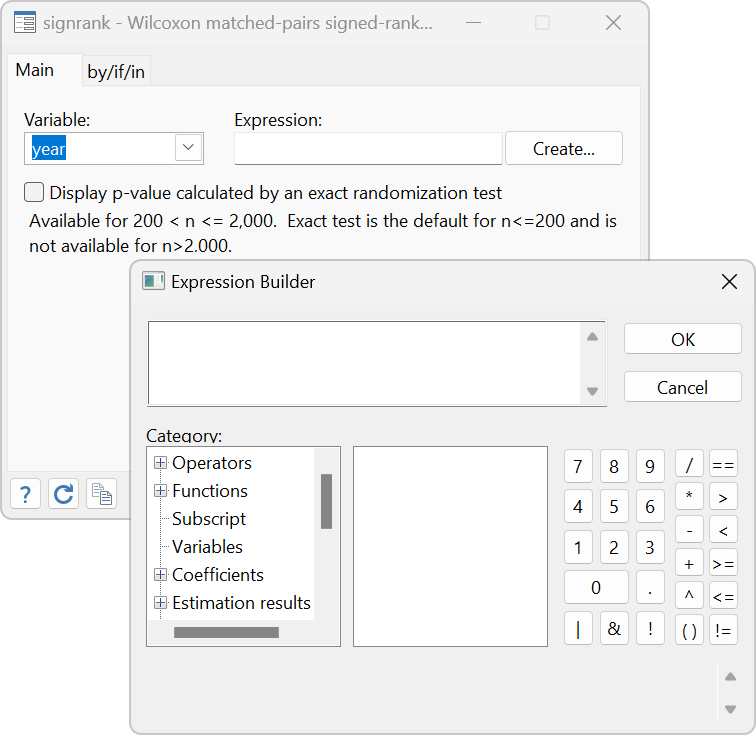NONPARAMETRIC TESTS

• Sign test
• Wilcoxon signed-rank test• Wilcoxon rank-sum (Mann–Whitney) test
• Equality of medians
• Kruskal–Wallis test
• Kolmogorov–Smirnov test
• Binomial probability test
• Chi-squared tests for association
• Tests for trend
• Cochran–Armitage test
• Jonckheere–Terpstra test
• Linear-by-linear test
• Cuzick’s test
• Exact p-values for tests
• One- and two-sample tests of proportions
• Test for random order
• McNemar’s chi-squared test
• Equality of survivor functions
• Equality of ROC areas
• Equality of ROC area against a standard ROC curve
• CUSUM
• Interrater agreement

QUANTILE REGRESSION

• Median regression
• Least absolute deviations regression
• User-specified quantiles
• Koenker and Bassett and Huber sandwich standard errors with many choices of bandwidth methods and kernels
• Simultaneous quantile regression
• Interquartile range regression

CENTILES AND CONFIDENCE INTERVALS

• Estimate percentiles
• Exact confidence intervals
• Optionally assume normality

RESAMPLING AND SIMULATIONS

• Bootstrap sampling and estimation
• Sampling with replacement
• Monte Carlo simulations
• Permutation tests

SMOOTHING

• Univariate kernel density estimation
• Lowess smoothing
• Kernel-weighted local polynomial smoothing
• Robust nonlinear smoother

ROC ANALYSIS

• Plot ROC curves

NONPARAMETRIC KERNEL REGRESSION

• Multiple covariates supported
• Continuous covariates (8 kernels available)
• Discrete covariates (2 kernels available)
• Techniques
• local constant regression
• local linear regression
• Point estimates with SEs and CIs
• Derivative estimates with SEs and CIs
• Automatic optimal bandwidth selection
• Interface to margins for advanced inference
• Estimates of population-averaged and subpopulation-averaged means and effects
• Fully conditional means and effects at

any specified values of the covariates

• Confidence intervals
• Tests
• Graphs via marginsplot

NONPARAMETRIC SERIES REGRESSION

• Multiple covariates supported
• Continuous covariates
• Discrete covariates
• Series approximation with series
• B-splines basis
• Piecewise polynomial splines
• Polynomials
• Semiparametric regression models
• Optimal knot and polynomial selection
• cross-validation
• generalized cross-validation
• AIC
• BIC
• Mallows’s Cp
• Interface to margins
• Estimates of population and subpopulation means and effects
• Fully conditional means and effects
• Confidence intervals
• Graphs via marginsplot

SPLINE BASIS FUNCTION GENERATION

• B-spline
• Piecewise polynomial splines
• Restricted cubic splines
• Flexible knot selection
• number of knots
• knot list
• knots matrix

NONPARAMETRIC CORRELATION COEFFICIENTS

• Spearman’s rank order
• Kendall’s rank order

SURVIVAL ANALYSIS

• Kaplan–Meier curves
• Nelson–Aalen curves
• Logrank and other tests of equality

MULTIVARIATE ANALYSIS

• KNN discriminant analysis

CAUSAL INFERENCE/TREATMENT EFFECTS

• Nearest-neighbor matching
• Propensity-score matching